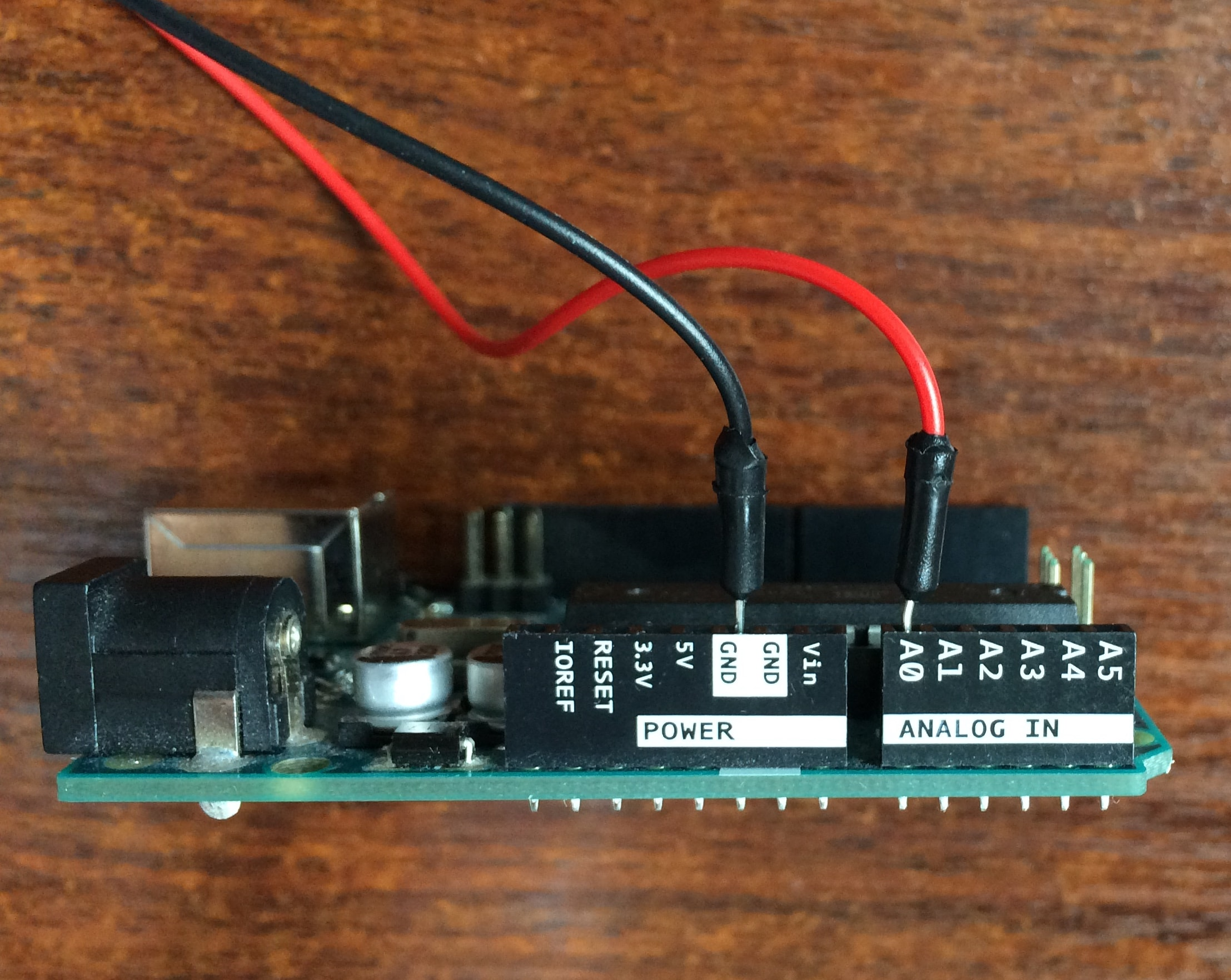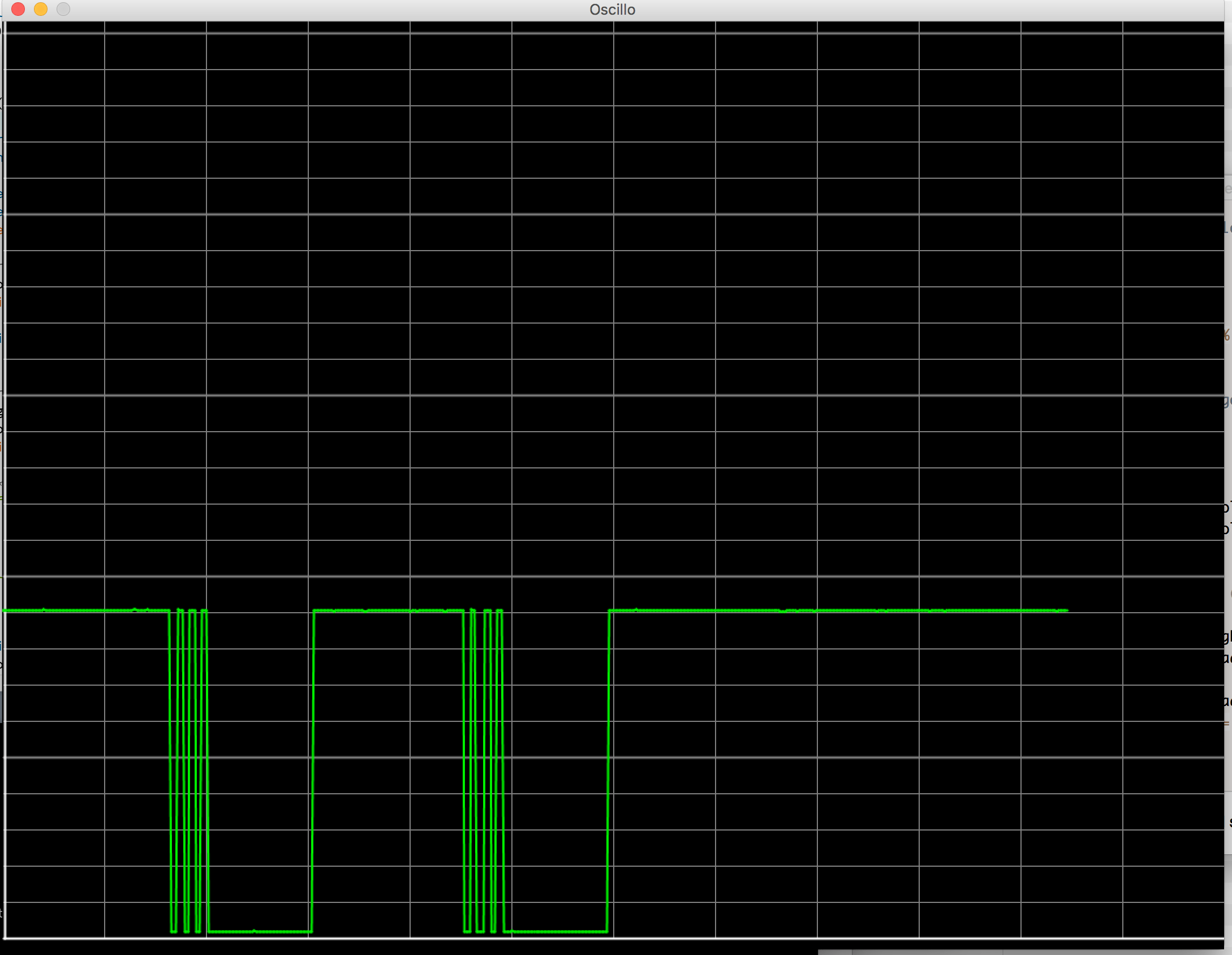# Build an oscilloscope with an Arduino

## Really cheap, really easy

Posted by Alexandre on April 5, 2016 and updated on September 1, 2016

Update (01/09/2016): New version here

Let’s say you bought an Arduino and a starter kit, but you don’t own (yet!) a lot of tools, like a multimeter or an oscilloscope. And yet, you need to read a tension to make sure your circuit is working properly. How do you do that?

You’ll only need:

• 1x Arduino

There are some limitations though:

You can only measure voltage between 0V and 5V. The `analogRead()` function of the Arduino will run in approximately 100 microseconds: the sampling rate cannot be above 10kHz.

## The Arduino Program

This part is really straightforward. The idea is to acquire the signal on the analog pin of the Arduino and send it on the serial communication.

``````/*
* This program will run on the Arduino. It's role is to acquire the signal
*/
int measurePin=0; /* We use A0 pin as input */

int voltage=0; /* Container of the analog reading */
int period=20; /* Delay between two measures (in ms) */

void setup()
{
Serial.begin(19200); /* Initialization of serial communication */
pinMode(measurePin, INPUT);
}

void loop()
{
Serial.println(voltage);
delay(period);
}
``````

Put a wire in the A0 analog port and another to the ground## The Processing program

Processing have a UI very similar to Arduino IDE so you shouldn’t be disorientated. You can simply type in a program and click on the Run button.

This program reads the data on the serial communication and render it as a graph.

``````import processing.serial.*;
Serial serialConnexion;
int voltage=0;
int border = 10;
int windowTime=12000; /* time span of the screen (in ms) */
int x=0;
int y=0;

void setup()
{
size(1080, 820); /* Screen size */
// Take the serial portname
// change the index according to the order of your ports
String portName = Serial.list();
serialConnexion = new Serial(this, portName, 19200); /* Create the connexion */
println("Connected to "+portName);
grid();

}

void grid()
{
background(0); // white background
smooth(); // smooth drawings

strokeWeight(1);
stroke(128,128,128); // color of grid (gray)
double timeNotch, voltageNotch;

// Vertical lines for the time: 1s scale
timeNotch = (double)width / (double)windowTime * 1000.0;
for (int i = (int)timeNotch; i <= width; i+=(int)timeNotch)
{
line(i, 0, i, height - border);
}

// Horizontal lines for the voltage: 0.2V scale
voltageNotch = ((double)height - (double)(border * 2.0)) * 0.2 / 5.0;
int counter = 0;
for (int j =(int)voltageNotch; j <= height - border; j+= (int)voltageNotch)
{
/* Every volt, we draw a large line */
if (counter % 5 == 4)
{
strokeWeight(2);
}
else
{
strokeWeight(1);
}
line(1, height - border - j, width, height - border - j);
counter++;
}

strokeWeight(2); // width of lines
stroke(255,255,255); // color of the axis (white)
line(2, 0, 2, height - border); // Vertical ligne
line(1, height - border, width, height - border); // Horizontal line

strokeWeight(2);
stroke(0,240,0);   // color of line (green)
}
/* Main drawing loop */
void draw()
{

int oldx=x;
x=(millis() % windowTime) * width / windowTime;
if (oldx>x)
{
oldx=0; /* go back to the start of the screen */
grid();
}

int oldy=y;
y=int(map(voltage, 0, 1023, height - border, border)); /* resize y between 20 and 680 */
line(oldx, oldy, x, y); /* draw the line */
}

void serialEvent (Serial serialConnexion)
{
{“L” led voltage when the reset button is pressed (on another arduino)